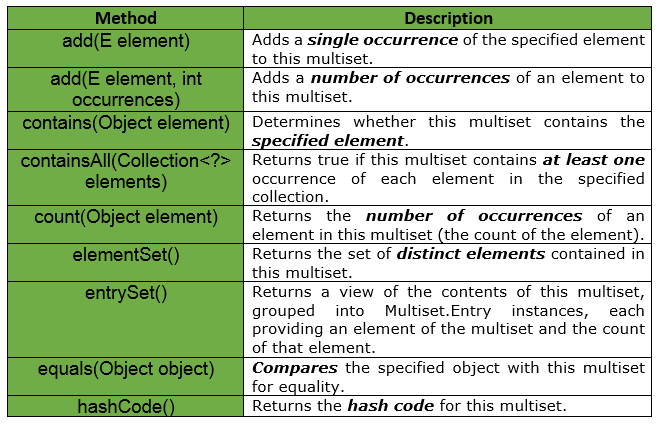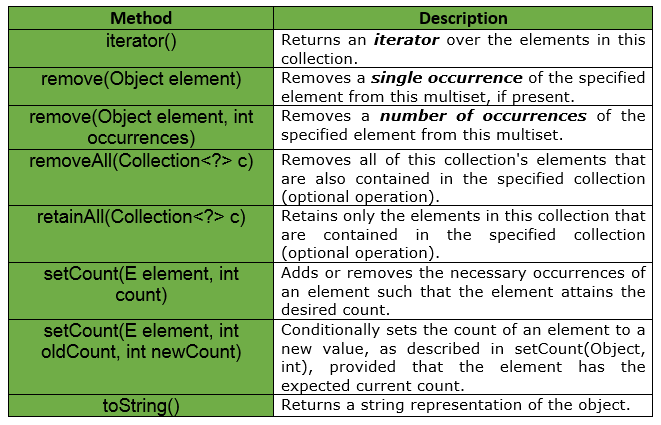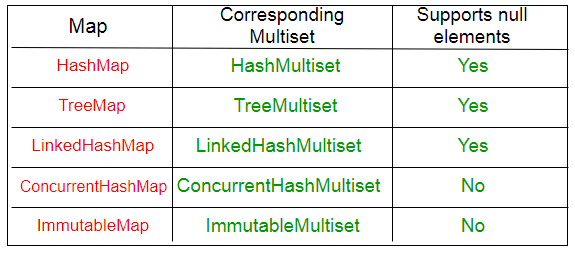# Multiset Interface | Guava | Java

Introduction : Multiset is a collection that supports order-independent equality, like Set, but may have duplicate elements. We might think that a Multiset is a List then, but it is not so.

• Lists can hold duplicates of the same object, and Lists are always ordered.
• Sets can’t hold duplicates, and there’s no guarantee of order by the Set interface.

So a Multiset occupies a sort of grey area between a List and a Set. Duplicates allowed, but no guaranteed order. A multiset is also sometimes called a bag. In multisets, as in sets and in contrast to tuples, the order of elements is irrelevant in multisets. For example : The multisets {a, a, b} and {a, b, a} are equal.

There are two main ways of looking at Multiset :

• This is like an ArrayList<E> without an ordering constraint i.e, ordering does not matter.
• This is like a Map<E, Integer>, with elements and counts.

Important Points :

• Multiset is a collection that supports order-independent equality, like Set, but may have duplicate elements.
• Elements of a multiset that are equal to one another are referred to as occurrences of the same single element.
• The total number of occurrences of an element in a multiset is called the count of that element.
• Since the count of an element is represented as an int, a multiset may never contain more than Integer.MAX_VALUE occurrences of any one element.
• A multiset uses Object.equals(java.lang.Object) to determine whether two instances should be considered “the same, ” unless specified otherwise by the implementation.
• A Multiset<E> has elements with positive counts only. No element can have negative counts, and values with count 0 are considered to not be in the multiset. They do not appear in the elementSet() or entrySet() view.
• multiset.size() returns the size of the collection, which is equal to the sum of the counts of all elements. For the number of distinct elements, use elementSet().size(). For example, add(E) increases multiset.size() by one.
• multiset.iterator() iterates over each occurrence of each element, so the length of the iteration is equal to multiset.size().
• Multiset supports adding elements, removing elements, or setting the count of elements directly. setCount(element, 0) is equivalent to removing all occurrences of the element.
• multiset.count(element) for an element not in the multiset always returns 0.

Declaration : The declaration for com.google.common.collect.Multiset interface is as below :

```@GwtCompatible
public interface Multiset
extends Collection
```Example : We know that if two equal elements are added to java.util.Set, then the second element will be discarded.

 `// Java implementation to show if 2 ` `// equal elements are added to ` `// java.util.Set, then the 2nd element ` `// will be discarded ` ` `  `import` `java.util.Set; ` `import` `java.util.HashSet; ` ` `  `// Set of Strings ` `Set set = ``new` `HashSet(); ` ` `  `// Adding elements to the set ` `set.add(``"Geeks"``); ` `set.add(``"Geeks"``); ` ` `  `System.out.println(set); ` ` `  `// The output will be [Geeks] `

But, if we use Guava’s Multiset, then the duplicate element will not be discarded. See the code below for implementation :

 `// Java implementation to show if 2 ` `// equal elements are added to ` `// Multiset, then the 2nd element ` `// will not be discarded ` ` `  `import` `com.google.common.collect.HashMultiset; ` `import` `com.google.common.collect.Multiset; ` ` `  `// Multiset of String ` `Multiset multiset = HashMultiset.create(); ` ` `  `// Adding elements to the set ` `multiset.add(``"Geeks"``); ` `multiset.add(``"Geeks"``); ` ` `  `System.out.println(multiset); ` ` `  `// The output will be [Geeks X 2] `

Below given are some other methods provided by Multiset Interface of Guava :Implementations :
Guava provides many implementations of Multiset, which roughly correspond to JDK map implementations.Example for Set :

 `// Java code to show implementation ` `// of a Set ` `import` `java.util.*; ` ` `  `import` `com.google.common.collect.HashMultiset; ` `import` `com.google.common.collect.Multiset; ` ` `  `class` `GuavaTester { ` ` `  `    ``// Driver code ` `    ``public` `static` `void` `main(String args[]) ` `    ``{ ` ` `  `        ``// Creating a Set of Strings ` `        ``Set set = ``new` `HashSet(); ` ` `  `        ``// Adding elements to set ` `        ``set.add(``"Geeks"``); ` `        ``set.add(``"for"``); ` `        ``set.add(``"Geeks"``); ` `        ``set.add(``"for"``); ` `        ``set.add(``"GeeksforGeeks"``); ` `        ``set.add(``"Geeks"``); ` `        ``set.add(``"GeeksforGeeks"``); ` `        ``set.add(``"Geeks"``); ` ` `  `        ``// printing the total size of the set ` `        ``System.out.println(``"Total Size is : "` `+ set.size()); ` ` `  `        ``// print the occurrence of each element ` `        ``System.out.println(``"Occurrences of Geeks are : "` `+ Collections.frequency(set, ``"Geeks"``)); ` ` `  `        ``System.out.println(``"Occurrences of for are : "` `+ Collections.frequency(set, ``"for"``)); ` ` `  `        ``System.out.println(``"Occurrences of GeeksforGeeks are : "` `+ Collections.frequency(set, ``"GeeksforGeeks"``)); ` `    ``} ` `} `

Output :

```Total Size is : 3
Occurrences of Geeks are : 1
Occurrences of for are : 1
Occurrences of GeeksforGeeks are : 1
```

Example for Multiset :

 `// Java code to show implementation ` `// of a Multiset ` `import` `java.util.*; ` ` `  `import` `com.google.common.collect.HashMultiset; ` `import` `com.google.common.collect.Multiset; ` ` `  `class` `GuavaTester { ` ` `  `    ``// Driver code ` `    ``public` `static` `void` `main(String args[]) ` `    ``{ ` ` `  `        ``// Creating a Multiset of Strings ` `        ``Multiset multiset = HashMultiset.create(); ` ` `  `        ``// Adding elements to multiset ` `        ``multiset.add(``"Geeks"``); ` `        ``multiset.add(``"for"``); ` `        ``multiset.add(``"Geeks"``); ` `        ``multiset.add(``"for"``); ` `        ``multiset.add(``"GeeksforGeeks"``); ` `        ``multiset.add(``"Geeks"``); ` `        ``multiset.add(``"GeeksforGeeks"``); ` `        ``multiset.add(``"Geeks"``); ` ` `  `        ``// printing the total size of the multiset ` `        ``System.out.println(``"Total Size is : "` `+ multiset.size()); ` ` `  `        ``// print the occurrence of each element ` `        ``System.out.println(``"Occurrences of Geeks are : "` `+ multiset.count(``"Geeks"``)); ` ` `  `        ``System.out.println(``"Occurrences of for are : "` `+ multiset.count(``"for"``)); ` ` `  `        ``System.out.println(``"Occurrences of GeeksforGeeks are : "` `+ multiset.count(``"GeeksforGeeks"``)); ` `    ``} ` `} `

Output :

```Total Size is : 8
Occurrences of Geeks are : 4
Occurrences of for are : 2
Occurrences of GeeksforGeeks are : 2
```

My Personal Notes arrow_drop_upIn love with a semicolon because sometimes i miss it so badly)

If you like GeeksforGeeks and would like to contribute, you can also write an article using contribute.geeksforgeeks.org or mail your article to contribute@geeksforgeeks.org. See your article appearing on the GeeksforGeeks main page and help other Geeks.

Please Improve this article if you find anything incorrect by clicking on the "Improve Article" button below.

Article Tags :
Practice Tags :

1

Please write to us at contribute@geeksforgeeks.org to report any issue with the above content.